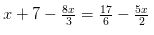# Solve the following linear equations: x + 7 - 8x/3 = 17/6 - 5x/2

Solve the following linear equations: